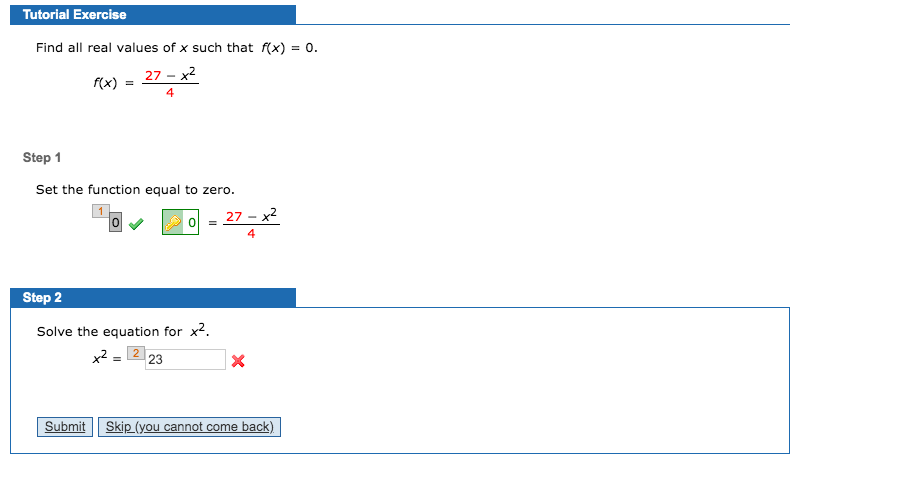# Tutorial ExerciseFind all real values of x such that f(x) = 0.27 - x2f(x)Step 1Set the function equal to zero.27 - x2Step 2Solve the equation for x2.x2 = 2 23Skip (you cannot come back)Submit

Question
26 viewshelp_outlineImage TranscriptioncloseTutorial Exercise Find all real values of x such that f(x) = 0. 27 - x2 f(x) Step 1 Set the function equal to zero. 27 - x2 Step 2 Solve the equation for x2. x2 = 2 23 Skip (you cannot come back) Submit fullscreen
check_circle

Step 1

### Want to see the full answer?

See Solution

#### Want to see this answer and more?

Solutions are written by subject experts who are available 24/7. Questions are typically answered within 1 hour.*

See Solution
*Response times may vary by subject and question.
Tagged in

### Other